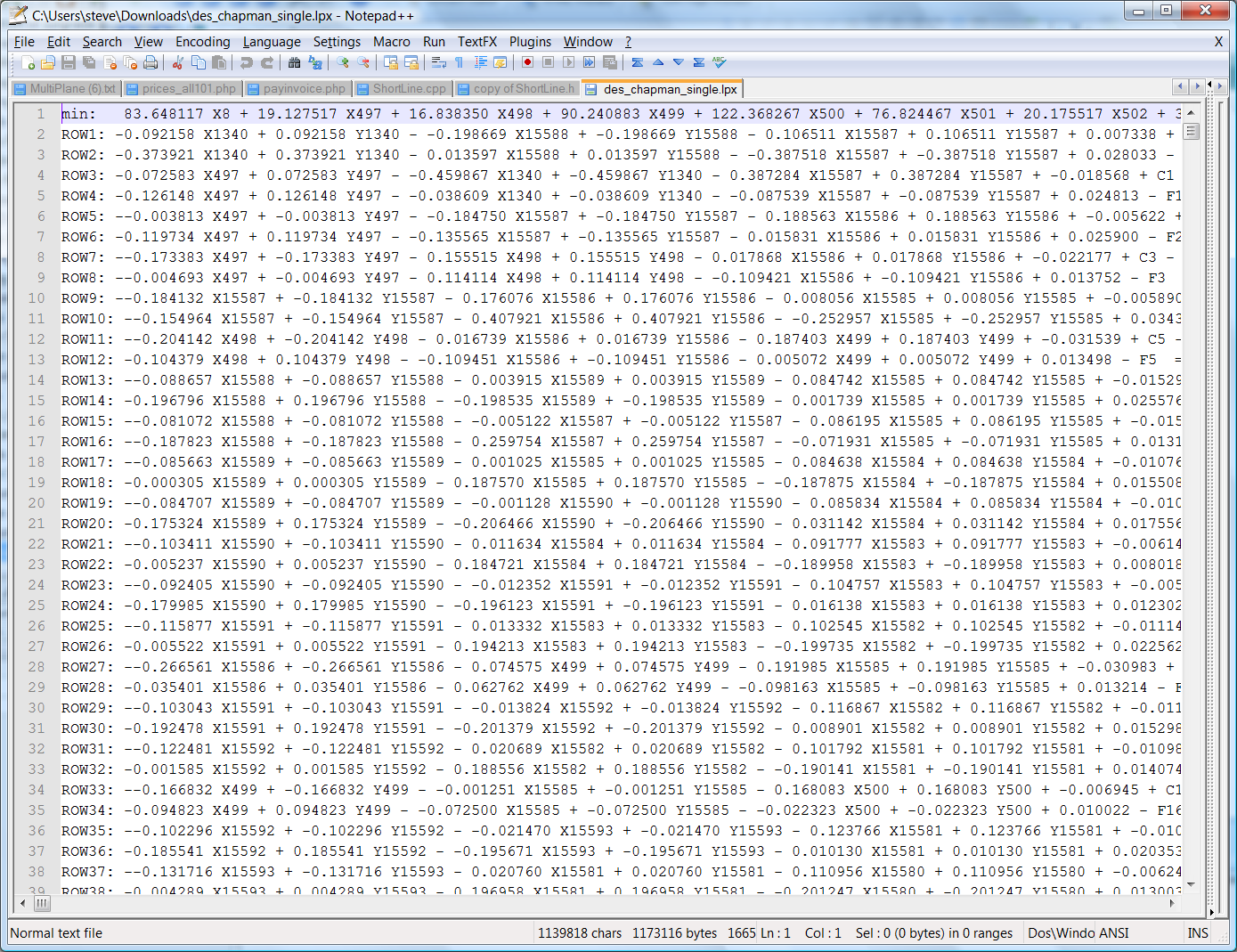# Mathematical Basis for Algorithms

What follows is the basis of how our algorithms work.

Our design is loosely based on a paper written in 1973; "Land Forming Design By Linear Programming" written by "Sowell, Shih and Kriz". The paper is available from Transactions of the ASCE and published in 1973.

Linear Programming simply defines an efficient method of creating a solution where all the mathematics involved is linear. For example a straight line is linear while a parabola is nonlinear and could not be solved using these methods. You then create a series of constraining equations which define the mathematical model. In this case our preferred design surface.

Ezigrade gives you the option of solving constrained grade sections using a grid based method. This is the default solution. The job is split into grids and the grid points are solved to minimize the sum of the cuts. In both directions if necessary if constrains are given in both main grade and cross grade directions. The advantage of the grid based solution is that the amount and accuracy of processing can be controlled by controlling the grid size. Grid size bigger - less processing for initial designs. When you are happy with the design you can increase the grid density for a more accurate design.

Ezigrade also gives you a LP solution based on the natural surface triangles. In this solution Ezigrade picks up the points used in the natural surface triangles. LP needs either a minimum or maximum to solve. In our rendition we solve the minimum of the sum of the cut distances for each of the natural surface points. We weight each point depending on the size of the triangle it is contained by. In this way we are approximating solving the total volume. Please be aware the actual equation for the surface volumes is not linear so can't be used directly.

In the LP model we then create a series of constraining equations based on the cut and fills of each line created along the edge of the triangles. We also add in some additional constraints. In the screen shot below we can see a typical solution. Each X and Y represents a cut and fill at each point.

This design is then added into a generic Linear Programming solution.

In our case we use lp_solve, which is a general purpose Linear Programming solution. I also suspect that Agform-3d uses the same system as they include a file lpsolverdll.dll with there solution.

Here is a typical solution file below:## Smoothing Solution:

Ezigrade has a solution that gives an "optimal" smoothing solution for the whole surface. We believe our solution is different to some of our competitors. As far as I am aware the common solution is to specify a variable that limits the change in grade between points along the grid. For example in Agform3D they have a slope-rate constraint variable which is effected along the row and columns. However being grid based I am assuming any changes diagonally are ignored. This additional constraint can be added into the Linear Programming equations. Ezigrade uses a different method based on Least Squares which simply works based on the difference between points. Feedback we have received indicates our completed fields have a more natural smoothed look; which I must admit is very hard to quantify.

In our solution we specify a distance. You can think of this sampling distance as starting at our sampling point and drawing a circle based on the sampling distance. All the points contained within the circle are used in the least squares solution.

Using these values we find the new height of the sampling point based on the weighting of all the points within the sampling distances. We repeat this for the entire solution and set all the new design heights appropriately.

## Water always flows to the edge of the job:

This is a field onto itself with the GIS community; and there are a lot of different academic papers in the literature. To access these resources you can google "Depression Filling" which shows the depth of this subject. It is also common in the literature to pass a low pass filter over the surface first (ie smoothing off ridges and filling valleys etc) before running the algorithm. We have based our work on these existing algorithms.

There are a couple of existing GIS software solutions that can perform this operation. For example "WhiteBox GAT" and ESRI "Arc GIS". However obviously we prefer that you use Ezigrade as we create an output directly that you can use on your favorite grading machine.

 TODO: Change the footer's content in Project Settings : HTML (CHM) Export : Setup HTML Template and Layout